# torque

## Torque

• Consider two forces F1 and F2 having equal magnitude and opposite direction acting on a stick placed on a horizontal table as shown below in the figure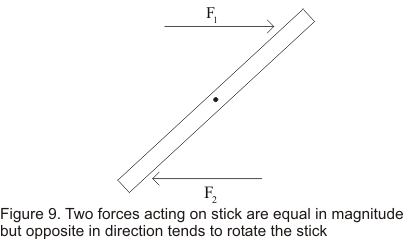• Here note that line of action of forces F1 and F2 is not same .So they tend to rotate the stick in clockwise direction
• This tendency of the force to rotate an object about some axis is called torque
• Torque is the rotational counterpart of force. torque tends to rotate an body in the same way as force tends to change the state of motion of the body
• Figure below shows a rigid body pivoted at point O so that point O is fixed in space and the body is free to rotate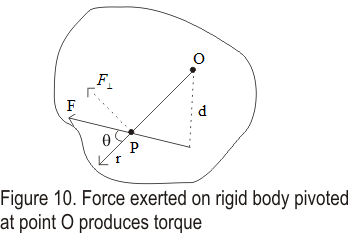• Let P be the point of application of force. This force acting at point P makes an angle θ with the radius vector r from point O to P
• This force F can be resolved into two components
F=Fsinθ
F||=Fcosθ
as they are perpendicular and parallel to r
• Parallel component of force does not produce rotational motion of body around point O as it passes through O
• Effect of perpendicular components producing rotation of rigid body through point O depends on magnitude of the perpendicular force and on its distance r from O
• Mathematically ,torque about point O is defined as product of perpendicular component of force and r i.e.
τ=Fr=Fsinθr=F(rinθ)=Fd              ---(18)
where d is the perpendicular distance from the pivot point ) to the line of action of force F
• Quantity d=rinθ is called moment arm or liner arm of force F .If d=0 the there would be no rotation
• Torque can either be anticlockwise or clockwise depending on the sense of rotation it tends to produce
• Unit of torque is Nm
• Consider the figure given below where a rigid body pivoted at point O is acted upon by the two force F1 and F2
• d1 is the moment arm of force F1 and d2 is the moment arm of force F2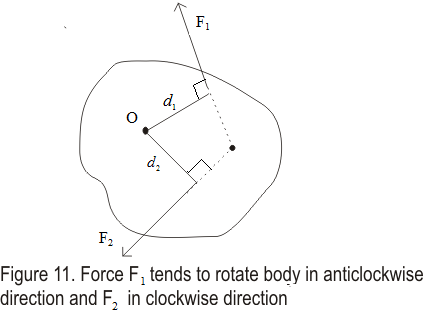• Force F2 has the tendency to rotate rigid body in clockwise direction and F1 has the to rotate it in anti clockwise direction
• Here we adopt a convention that anticlockwise moments are positive and clockwise moment are negative
• hence moment τ1 of force F1 about the axis through O is
τ1=F1d1
And that of force F2 would be
τ2=-F2d2
• Hence net torque about O is
τtotal= τ1+ τ2
=F1d1-F2d2
• Rotation of the body can be prevented if
τtotal=0
or τ1=-τ2
• We earlier studied that when a body is in equilibrium under the action of several coplanar forces ,the vector sum of these forces must be zero i.e.
ΣFx=0 and ΣFy=0
• we know state our second condition for static equilibrium of rigid bodies that is
" For static equilibrium of rigid body net torque in clockwise direction must be equal to net torque in anticlockwise direction w.r.t some specified axis i.e.
Στ=0
"
• Thus for static equilibrium of an rigid body
i) The resultant external force must be zero
ΣF=0
ii) The resultant external torque about any point or axis of rotation must be zero i.e.
Στ=0

## Torque in Vector Notation

• The turning effect of the force about the axis of rotation is called the moment of force or torque.
• In rotational motion torque has same importance as that of force in the linear motion.
• Torque due to a force F is measured as a vector product of force F and position vector r of line of action of force from the axis of rotation.
• We already know that torque is denoted by letter τ.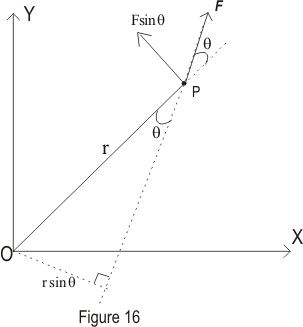• If F is the force acting on the particle and r is the position vector of particle with respect to constant point then the torque acting on the particle is given by
τ=r×F                         (8)
• FRom equation 8 magnitude or resultant of torque is given by
|τ|=rfsinθ                         (9)
where θ is the angle between r and F.
• From equation 9 if θ=900 this means r is perpendicular to F then,
• FRom equation 8 magnitude or resultant of torque is given by
|τ|=rF
and if θ=00 this means r is parallel to F then,
|τ|=0
• Unit of torque is Dyne-cm or Newton-m

## (9) Torque and angular acceleration

• While discussing and defining torque or moment of force ,we found that necessary condition for a body not to rotate is that resultant torque about any point should be zero
• However this condition is necessary but not sufficient for a rigid body to be static for example in absence of resultant torque a body once set in rotation will continue to rotate with constant angular velocity
• Analogous to translation motion when torque acts on a rigid body rotating about a point with constant angular velocity then angular velocity of the body does not remain constant but changes with angular acceleration α which is proportional to the externally applied torque
• Consider a force Fi acting on the ith particle of mass mi of the rigid body pivoted about an axis through point O as shown below in the figure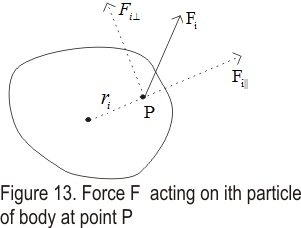• This force Fi as discussed earlier has two components one parallel to the radius vector ri and one perpendicular to the ri
• Component of force parallel to radius vector does not have any effect on the rotation of the body
• Component of force Fi perpendicular does affect the rotation of the body and produces torque about point O through which the body is pivoted which is given by
τi=Fi⊥ri ---(21)
• if Fi⊥ is the resultant force acting on the ith particle ,then from newton's second law of motion
Fi⊥=miai⊥ = miriα ----(22)
where ai⊥ is the tangential acceleration of the body
• From equation (21) and (22)
τi=miri2α
And taking sum over all the particles in the body we have
∑τi=∑(miri2α)=α∑(miri2) ---(23)
as angular acceleration is same for all the particles of the body
• we know that
∑(miri2) =I
where I is the moment of inertia of the rigid body .Hence in terms of moment of inertia equation 23 becomes
∑τ=Iα                      ---(24)
we have denoted resultant torque acting on the body ∑τsub>i as ∑τ
• Both the torque and angular acceleration are vector quantities so in vector form
τ=Iα                ---(25)
• Alternatively equation (24) which is rotational analogue of Newton second law of motion ( ∑F=ma) can be written as
τ=Iα = I(dω/dt)=d(Iω)/dt                      ---(26)
which is similar to the equation
F=d(mv/dt=dp/dt
where p is the Linear Momentum
• The quantity Iω is defined as the angular momentum of the system of particles
Angular momentum =Iω
L=Iω
• From equation 26 we see that resultant torque acting on a system of particles equal to the rate of change of the angular momentum
τ=dL/dt